# Mass Metric Units

##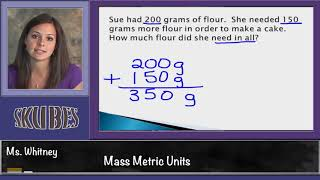By Skubes ed

Measure and estimate liquid volumes and masses of objects using standard units of grams (g), kilograms (kg), and liters (l)# Mass problems | Measurement and geometry

##By Khan Academy

Solve word problems involving mass. ï¿½ï¿½ï¿½ï¿½ï¿½ï¿½ï¿½ï¿½ï¿½ï¿½ï¿½ï¿½Estimate the mass of items.# Intuition for grams | Measurement and geometry

##By Khan Academy

In this video, we think about what what mass really means. We also examine the masses of real objects in grams and kilograms.# Vacation or Conservation (Of Mass): Crash Course Kids #23.1

##By Crash Course Kids# Units of Measure | Good To Know | WSKG

##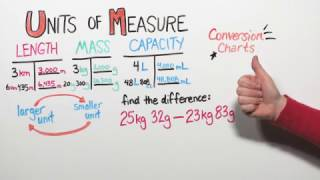By WSKG Public Media

metric system to measure length, mass, and capacity.# Rotational Dynamics - Basic Introduction

##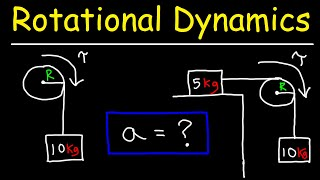By The Organic Chemistry Tutor

This physics video tutorial provides a basic introduction into rotational dynamics. It explains how to calculate the acceleration of a hanging mass attached to a rotating pulley. Examples include pulleys with two hanging masses (atwood machine) and inclined planes with pulleys and kinetic friction.# 3rd Grade Lesson 10.8: Estimate and Measure Mass

##By ValerieWilluweit# [3.MD.2] Mass/Volume Measurements

##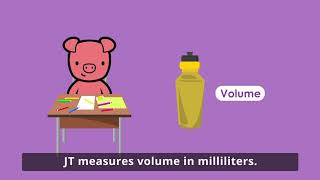By Freckle by Renaissance# Measuring Volume in Metric Units

##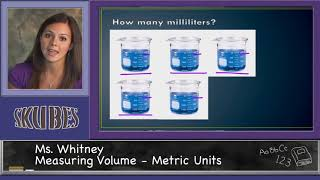By Skubes ed

Measure and estimate liquid volumes and masses of objects using standard units of grams (g), kilograms (kg), and liters (l)# Measuring Volume using Customary Units

##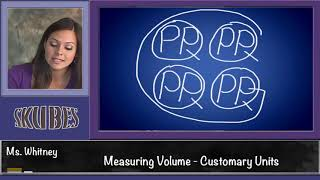By Skubes ed

Measure and estimate liquid volumes and masses of objects using standard units of grams (g), kilograms (kg), and liters (l)# Converting Units Using Dimensional Analysis

##By HowCanIChem

This is an example of how to convert units. In this specific video, we go from years to seconds using dimensional analysis.# Types of Communication

##By Krista Price

Krista Price outlines five types of communication: intrapersonal, interpersonal, group, mass, and formal public speaking. She gives examples of each type.# Colligative Properties - Boiling Point Elevation, Freezing Point Depression & Osmotic Pressure

##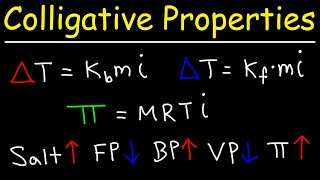By The Organic Chemistry Tutor

This chemistry video tutorial provides a basic introduction into colligative properties such as boiling point elevation, freezing point depression, osmotic pressure, and vapor pressure. It explains how to calculate the boiling point and freezing point of a solution as well as how to calculate the molar mass of a solute using osmotic pressure and freezing point depression.# Fractions and Line Plots

##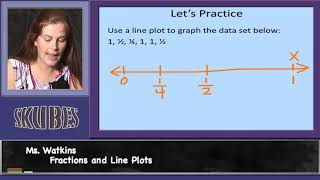By Skubes ed

Use the four operations to solve word problems involving distances, intervals of time, liquid volumes, masses of objects, and money, including problems involving simple fractions or decimals, and problems that require expressing measurements given in a larger unit in terms of a smaller unit.# (LEGO) Block Party: Crash Course Kids #23.2

##By Crash Course Kids# Gravity Compilation: Crash Course Kids

##By Lumos Learning

Maybe you'd like to just hear about one topic for a while. We understand. So today, let's just watch some videos about Gravity. We'll learn about why we don't fly off into space, what mass has to do with it, how does air resistance work, and why gravity is different on the moon. In this compilation,# Converting units word problems (metric)

##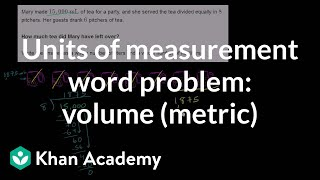By Khan Academy

Great word problem that get us thinking about volume. We'll need to express the answer in two different units of measurement.# Converting units word problems (US customary)

##By Khan Academy

Pints or gallons? Gallons or quarts? Confused? You won't be for long. This unit measurement word problem gets us thinking about moving between these units of volume.# Converting units word problems (US customary)

##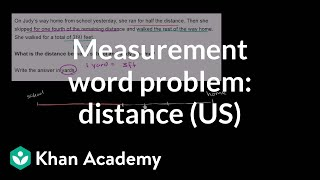By Khan Academy

We're applying what we've learned about converting units of measure in this word problem. We're also using arithmetic calculations. Let's give it a try!# Converting units (metrics)

##By Khan Academy

We're going to convert minutes into hours, but consider whether it is best to state the answer as a decimal or a mixed number. In truth, it doesn't make a difference...both are correct!